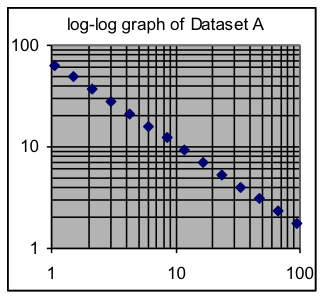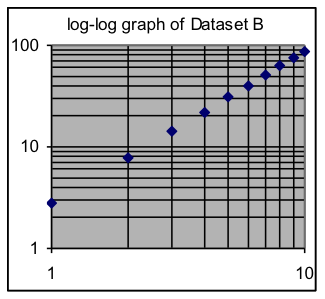## M1.07: Logarithmic Graphs Part II

Model-fitting using logarithms: It is possible, and sometimes useful, to fit models to the logarithms of the output values in a dataset, rather than to the output values themselves. For example, a linear model might be fit to the logarithm of data that has an exponential pattern. When this is done using minimization tools such as Solver, however, note that what is minimized is the standard deviation of the logarithms, not of the original values. In the case of logarithms, this has the same effect as minimizing relative standard deviation.

Log-log graphs: In situations where all x and y values are positive but there is a large dynamic range for both variables, it can help to use the logarithms of x values as well as for y values. A graph where this is done is called a “log-log” graph. On log-log graphs, straight lines indicate power-function relationships (in contrast to the exponential relationship indicated by straight lines on semi-log graphs). The slope of the line (0.5 on the graph of logarithms, below center) matches the power parameter in the model, and the intercept of the line (1.44 below) is the logarithm of the scale parameter 27.3.

Example 8: Use log-log graphs of each of these two datasets to determine whether their data follows a power-function pattern.Dataset A x y 1.06 63.95 1.50 48.52 2.12 36.80 2.99 27.91 4.22 21.17 5.96 16.06 8.42 12.18 11.90 9.24 16.80 7.01 23.74 5.32 33.53 4.03 47.36 3.06 66.90 2.32 94.50 1.76
 Dataset B x y 1 2.75 2 7.78 3 14.29 4 22.00 5 30.75 6 40.42 7 50.93 8 62.23 9 74.25 10 86.96

Using other functions of the output values: There are some situations in which it is convenient to apply other functions to the y data so that the result is close to a straight line. For example, the power function y = x2 can be made linear by taking the square root of the y values. This tactic was used more often before computers were widely available to fit nonlinear formulas, but such “linerarizations” are still used in some areas because people are very good at judging the straightness of lines and at using linear graphs for interpolation and extrapolation. You can tell when an axis has been transformed in this way because the gridlines of the graph will be non-uniform in spacing, like those of semi-log graphs.